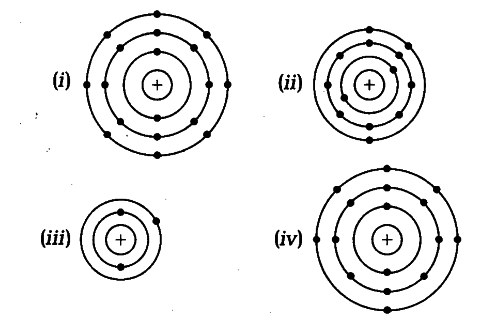# Find out the valency of atoms represented by the following figures

Find out the valency of atoms represented by the following figures.(i) Valency = 0 [•.‘number of valence electrons = 8]

(ii) Valency = 3 [•.'number of valence electrons =3]

(iii) Valency = 1 [•.'number of valence electron =1]

(iv) Valency = 2 [•.'number of valence electrons = 6]
[valency =8—6 = 2]